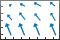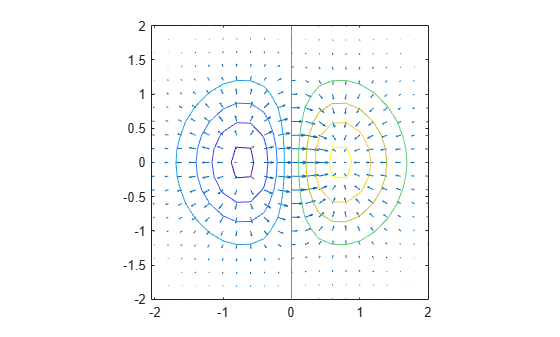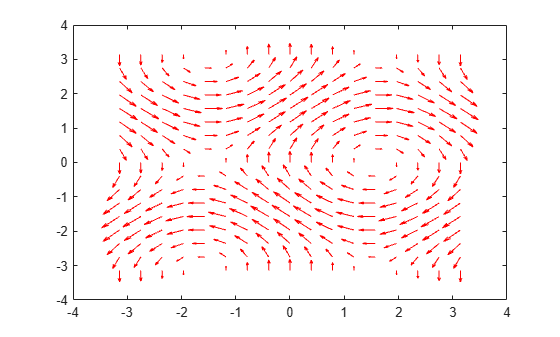# quiver

Quiver or vector plot

•## Syntax

``quiver(X,Y,U,V)``
``quiver(U,V)``
``quiver(___,scale)``
``quiver(___,LineSpec)``
``quiver(___,LineSpec,'filled')``
``quiver(___,Name,Value)``
``quiver(ax,___)``
``q = quiver(___)``

## Description

example

````quiver(X,Y,U,V)` plots arrows with directional components `U` and `V` at the Cartesian coordinates specified by `X` and `Y`. For example, the first arrow originates from the point `X(1)` and `Y(1)`, extends horizontally according to `U(1)`, and extends vertically according to `V(1)`. By default, the `quiver` function scales the arrow lengths so that they do not overlap.```
````quiver(U,V)` plots arrows with directional components specified by `U` and `V` at equally spaced points. If `U` and `V` are vectors, then the x-coordinates of the arrows range from 1 to the number of elements in `U` and `V`, and the y-coordinates are all 1.If `U` and `V` are matrices, then the x-coordinates of the arrows range from 1 to the number of columns in `U` and `V`, and the y-coordinates of the arrows range from 1 to the number of rows in `U` and `V`. ```

example

````quiver(___,scale)` adjusts the length of arrows: When `scale` is a positive number, the `quiver` function automatically adjusts the lengths of arrows so they do not overlap, then stretches them by a factor of `scale`. For example, a `scale` of 2 doubles the length of arrows, and a `scale` of 0.5 halves the length of arrows.When `scale` is `'off'` or `0`, such as `quiver(X,Y,U,V,'off')`, then automatic scaling is disabled. ```

example

````quiver(___,LineSpec)` sets the line style, marker, and color. Markers appear at the points specified by `X` and `Y`. If you specify a marker using `LineSpec`, then `quiver` does not display arrowheads. To specify a marker and display arrowheads, set the `Marker` property instead.```
````quiver(___,LineSpec,'filled')` fills the markers specified by `LineSpec`.```
````quiver(___,Name,Value)` specifies quiver properties using one or more name-value pair arguments. For a list of properties, see Quiver Properties. Specify name-value pair arguments after all other input arguments. Name-value pair arguments apply to all of the arrows in the quiver plot.```

example

````quiver(ax,___)` creates the quiver plot in the axes specified by `ax` instead of the current axes (`gca`). The argument `ax` can precede any of the input argument combinations in the previous syntaxes.```

example

````q = quiver(___)` returns a `Quiver` object. This object is useful for controlling the properties of the quiver plot after creating it.```

## Examples

collapse all

Load sample data that represents air currents over North America. For this example, select a subset of the data.

```load('wind','x','y','u','v') X = x(11:22,11:22,1); Y = y(11:22,11:22,1); U = u(11:22,11:22,1); V = v(11:22,11:22,1);```

Create a quiver plot of the subset you selected. The vectors `X` and `Y` represent the location of the base of each arrow, and `U` and `V` represent the directional components of each arrow. By default, the `quiver` function shortens the arrows so they do not overlap. Call `axis equal` to use equal data unit lengths along each axis. This makes the arrows point in the correct direction.

```quiver(X,Y,U,V) axis equal```By default, the `quiver` function shortens arrows so they do not overlap. Disable automatic scaling so that arrow lengths are determined entirely by `U` and `V` by setting the `scale` argument to `0`.

For instance, create a grid of `X` and `Y` values using the `meshgrid` function. Specify the directional components using these values. Then, create a quiver plot with no automatic scaling.

```[X,Y] = meshgrid(0:6,0:6); U = 0.25*X; V = 0.5*Y; quiver(X,Y,U,V,0)```Plot the gradient and contours of the function $z=x{e}^{-{x}^{2}-{y}^{2}}$. Use the `quiver` function to plot the gradient and the `contour` function to plot the contours.

First, create a grid of x- and y-values that are equally spaced. Use them to calculate z. Then, find the gradient of z by specifying the spacing between points.

```spacing = 0.2; [X,Y] = meshgrid(-2:spacing:2); Z = X.*exp(-X.^2 - Y.^2); [DX,DY] = gradient(Z,spacing);```

Display the gradient vectors as a quiver plot. Then, display contour lines in the same axes. Adjust the display so that the gradient vectors appear perpendicular to the contour lines by calling `axis equal`.

```quiver(X,Y,DX,DY) hold on contour(X,Y,Z) axis equal hold off```Create a quiver plot and specify a color for the arrows.

```[X,Y] = meshgrid(-pi:pi/8:pi,-pi:pi/8:pi); U = sin(Y); V = cos(X); quiver(X,Y,U,V,'r')```Create a grid of `X` and `Y` values and two sets of `U` and `V` directional components.

```[X,Y] = meshgrid(0:pi/8:pi,-pi:pi/8:pi); U1 = sin(X); V1 = cos(Y); U2 = sin(Y); V2 = cos(X); ```

Create a tiled layout of plots with two axes, `ax1` and `ax2`. Add a quiver plot and title to each axes. (Before R2019b, use `subplot` instead of `tiledlayout` and `nexttile`.)

```tiledlayout(1,2) ax1 = nexttile; quiver(ax1,X,Y,U1,V1) axis equal title(ax1,'Left Plot') ax2 = nexttile; quiver(ax2,X,Y,U2,V2) axis equal title(ax2,'Right Plot')```Create a quiver plot and return the quiver object. Then, remove the arrowheads and add dot markers at the base of each arrow.

```[X,Y] = meshgrid(-pi:pi/8:pi,-pi:pi/8:pi); U = sin(Y); V = cos(X); q = quiver(X,Y,U,V); q.ShowArrowHead = 'off'; q.Marker = '.';```## Input Arguments

collapse all

x-coordinates of the bases of arrows, specified as a scalar, a vector, or a matrix.

If `X` and `Y` are vectors and `U` and `V` are matrices, then `quiver` expands `X` and `Y` into matrices. In this case, `size(U)` and `size(V)` must equal `[length(Y) length(X)]`. For more information about expanding vectors into matrices, see `meshgrid`.

If `X` and `Y` are matrices, then `X`, `Y`, `U`, and `V` must be the same size.

y-coordinates of the bases of arrows, specified as a scalar, a vector, or a matrix.

If `X` and `Y` are vectors and `U` and `V` are matrices, then `quiver` expands `X` and `Y` into matrices. In this case, `size(U)` and `size(V)` must equal `[length(Y) length(X)]`. For more information about expanding vectors into matrices, see `meshgrid`.

If `X` and `Y` are matrices, then `X`, `Y`, `U`, and `V` must be the same size.

x-components of arrows, specified as a scalar, vector, or matrix.

If `X` and `Y` are vectors, then `size(U)` and `size(V)` must equal `[length(Y) length(X)]`.

If `X` and `Y` are matrices, then `X`, `Y`, `U`, and `V` must be the same size.

y-components of arrows, specified as a scalar, vector, or matrix.

If `X` and `Y` are vectors, then `size(U)` and `size(V)` must equal `[length(Y) length(X)]`.

If `X` and `Y` are matrices, then `X`, `Y`, `U`, and `V` must be the same size.

Line style, marker, and color, specified as a character vector or string containing symbols. The symbols can appear in any order. You do not need to specify all three characteristics (line style, marker, and color).

If you specify a marker using `LineSpec`, then `quiver` does not display arrowheads. To specify a marker and display arrowheads, set the `Marker` property instead.

Example: `'--or'` is a red dashed line with circle markers

Line StyleDescriptionResulting Line
`"-"`Solid line`"--"`Dashed line`":"`Dotted line`"-."`Dash-dotted lineMarkerDescriptionResulting Marker
`"o"`Circle`"+"`Plus sign`"*"`Asterisk`"."`Point`"x"`Cross`"_"`Horizontal line`"|"`Vertical line`"square"`Square`"diamond"`Diamond`"^"`Upward-pointing triangle`"v"`Downward-pointing triangle`">"`Right-pointing triangle`"<"`Left-pointing triangle`"pentagram"`Pentagram`"hexagram"`HexagramColor NameShort NameRGB TripletAppearance
`"red"``"r"``[1 0 0]``"green"``"g"``[0 1 0]``"blue"``"b"``[0 0 1]``"cyan"` `"c"``[0 1 1]``"magenta"``"m"``[1 0 1]``"yellow"``"y"``[1 1 0]``"black"``"k"``[0 0 0]``"white"``"w"``[1 1 1]`Arrow scaling factor, specified as a positive number or `'off'`. By default, the `quiver` function automatically scales the arrows so they do not overlap. The `quiver` function applies the scaling factor after it automatically scales the arrows.

Specifying `scale` is the same as setting the `AutoScaleFactor` property of the quiver object. For example, specifying `scale` as `2` doubles the length of the arrows. Specifying `scale` as `0.5` halves the length of the arrows.

To disable automatic scaling, specify `scale` as `'off'` or `0`. When you specify either of these values, the `AutoScale` property of the quiver object is set to `'off'` and the length of the arrow is determined entirely by `U` and `V`.

Target axes, specified as an `Axes` object. If you do not specify the axes, then the `quiver` function uses the current axes.

### Name-Value Arguments

Specify optional pairs of arguments as `Name1=Value1,...,NameN=ValueN`, where `Name` is the argument name and `Value` is the corresponding value. Name-value arguments must appear after other arguments, but the order of the pairs does not matter.

Before R2021a, use commas to separate each name and value, and enclose `Name` in quotes.

Example: `'Color','r','LineWidth',1`

Note

The properties listed here are only a subset. For a complete list, see Quiver Properties.

Width of arrow stem and head, specified as a scalar numeric value greater than zero in point units. One point equals `1/72` inch. The default value is `0.5` point.

Example: `0.75`

Arrowhead display, specified as `'on'` or `'off'`, or as numeric or logical `1` (`true`) or `0` (`false`). A value of `'on'` is equivalent to `true`, and `'off'` is equivalent to `false`. Thus, you can use the value of this property as a logical value. The value is stored as an on/off logical value of type `matlab.lang.OnOffSwitchState`.

• `'on'` — Display the vectors with arrowheads.

• `'off'` — Display the vectors without arrowheads.

Automatic scaling of arrow length, specified as `'on'` or `'off'`, or as numeric or logical `1` (`true`) or `0` (`false`). A value of `'on'` is equivalent to `true`, and `'off'` is equivalent to `false`. Thus, you can use the value of this property as a logical value. The value is stored as an on/off logical value of type `matlab.lang.OnOffSwitchState`.

• `'on'` — Scale the arrow length to fit within the grid-defined coordinate data and scale arrows so that they do not overlap. The `quiver` or `quiver3` function then applies the `AutoScaleFactor` to the arrow length.

• `'off'` — Do not scale the arrow lengths.

Scale factor, specified as a scalar. A value of `2` doubles the length of the arrows. A value of `0.5` halves the length of the arrows.

This property has an effect only if the `AutoScale` property is set to `'on'`.

Example: `2`

## Tips

To create a quiver plot using polar coordinates, first convert them to Cartesian coordinates using the `pol2cart` function.

## Version History

Introduced before R2006a